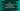# Dart optional positional, named and default parameters# Introduction :

Optional parameters in dart are used to mark any parameter optional i.e. you don’t have to pass value for that parameter. You can pass one value if you have, but your choice is optional. Optional parameters are categorised into three types : positional, named, and default.

## Optional positional :

Square bracket [] is used for optional positional parameters. Put all parameters in a square bracket to make them positional. It’s syntax is :

``````function ([ ...optional_param ]){

}``````

For example :

``````printAll(String p1, [String p2, String p3]) {
print("p1 = \$p1 || p2 = \$p2 || p3 = \$p3");
}

main() {
printAll("one");
printAll("one", "two");
printAll("one", "two", "three");
}``````

It will print the below output :

``````p1 = one || p2 = null || p3 = null
p1 = one || p2 = two || p3 = null
p1 = one || p2 = two || p3 = three``````

printAll function has three parameters. The first parameter p1 is a required parameter and the other parameters p2 and p3 are optional parameters.

If we don’t pass the value to an optional parameter, it will be null. So, always remember to do a null check if you are using optional parameters.

Positional optional parameters are assigned in the same order they are passed.

## Optional named :

For positional parameters, we need to pass the values in the same order as the parameters are defined in the function. Optional named parameters are similar to positional parameters but with a name. i.e. each parameter value can be assigned by its name.

The main advantage of optional named parameter is that we don’t have to maintain the order as they are defined. We can call them in any order.

Optional named parameters are placed inside curly braces {}. Below is the syntax for optional named parameter :

``````function ({ ...named_parameters }){

}``````

Let’s change the above example to use optional named parameters :

``````printAll(String p1, {String p2, String p3}) {
print("p1 = \$p1 || p2 = \$p2 || p3 = \$p3");
}

main() {
printAll("one");
printAll("one", p3: "two");
printAll("one", p3: "two", p2: "three");
}``````

We are not following the order as the parameters are defined here. Just use the name and pass them however you like.

For positional parameters, if you want only p1 and p3, you can’t do that without passing any value to p2. But with named parameters, you can pass any value to any parameter by using its name.

## Optional default :

We can assign default values to both positional and named parameters. The default value is null for a parameter if we are not assigning any value to that parameter. For example :

``````printAll(String p1, {String p2 = "p2", String p3 = "p3"}) {
print("p1 = \$p1 || p2 = \$p2 || p3 = \$p3");
}

main() {
printAll("one");
printAll("one", p3: "two");
printAll("one", p3: "two", p2: "three");
}``````

It will print :

``````p1 = one || p2 = p2 || p3 = p3
p1 = one || p2 = p2 || p3 = two
p1 = one || p2 = three || p3 = two``````

As you can see, it prints the default values if we are not passing any value for that parameter.